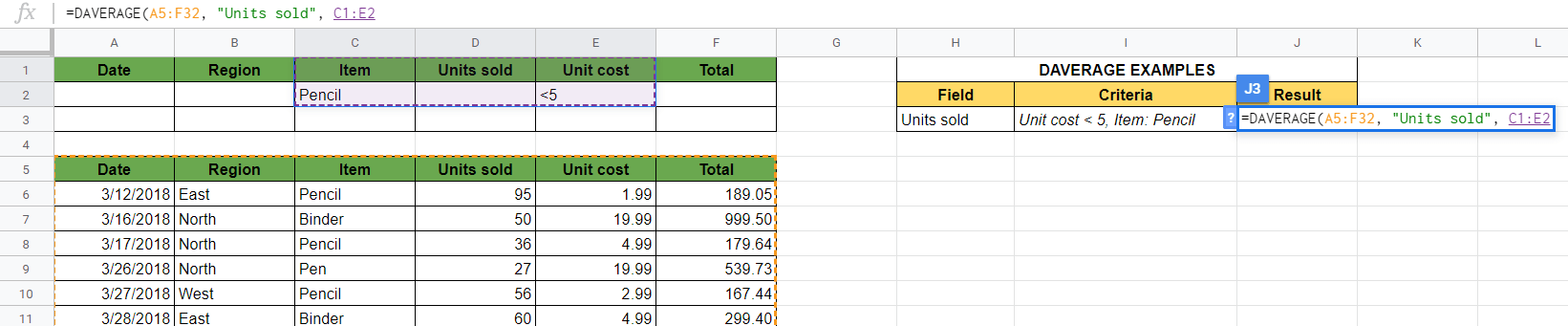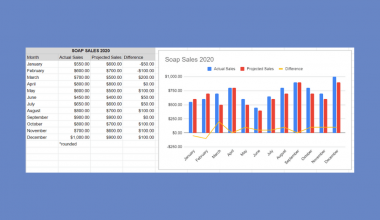# How to Use DAVERAGE Formula in Google Sheets

The DAVERAGE formula in Google Sheets is useful to get the average of values that meet specific criteria.

The `DAVERAGE` function is a database aggregation function, so the ‘D’ stands for ‘Database’.

It’s an average function with additional criteria. Its purpose is to calculate the average of only those items that match the criteria we define.

Let’s take an example.

Say you have a list of the office products you sold. ✏️

You have information about the sold products on your sheet. It has the regions where you traded, the type of products, the unit price, the total price paid, and so on.

You might want to calculate the average of some specific rows. For example, the average price of purchases only in the Northern region. Or count the average number of units sold only when the total price was higher than 500 dollars.

So how do we do that?

These are average calculations, but with one or more conditions by which to filter the data set.

The `DAVERAGE` function calculates the average in a specific database for a subset of data that matches our criteria.

Let’s dive right into some examples where we will deal with actual values and see how we can write our own DAVERAGE formula in Google Sheets.

### The Anatomy of the DAVERAGE Function

The syntax of the function defines how we should work with it. In which case, the syntax of the `DAVERAGE` function is as follows:

`=DAVERAGE(database, field, criteria)`

Let’s understand what the DAVERAGE function and its attributes mean:

• `=` the equal sign is how we start just about any function in Google Sheets.
• `DAVERAGE` is our function. We will have to add the corresponding arguments `database` , `field` and `criteria` into it for it to work.
• `database` is the array or range containing all the data to consider. It must be structured in such a way that the first row contains the labels for each column’s values.
• `field` indicates which column of the database contains the values that we want to operate on. This argument may either be a text label matching a column header, or a numeric index indicating which column to consider.
• `criteria` is the range containing the criteria to filter the database values by before averaging.

It’s important when using the `DAVERAGE` formula that the dataset must be structured.

Structured data means that the data is arranged in a database table. Each column needs to have a label in the first row.

### ⚠️ A Few Notes to Make Your DAVERAGE Function Work Perfectly

1. It’s essential that there shouldn’t be any merged cells in the range. This is a requirement of structured data.
2. `DAVERAGE` formula averages only numbers. Otherwise, it returns #DIV/0! Error.
3. The labels must match in the database and criteria. The labels that don’t match will not find the criteria.
4. You must use all the three arguments of the function, but you can keep the `criteria` blank. In this case, the function calculates the average in the whole database and without criteria.

## A Real Example of Using DAVERAGE Formula

Let’s look at some examples of how to use the DAVERAGE formula in Google Sheets.This picture shows the history of the sold office products. We see that this is a structured data set, and each column has a label in their first rows starting from cell A5.

Above that, we can see the same labels with some rows below. This range has the criteria that we will use with the `DAVERAGE` formula.

### The DAVERAGE Formula With One Criteria

Let’s start with the basic case when we use one criterion by which we filter our rows.

We want to count the average of the total prices paid only in the North region.

In other words, we want to calculate the average of values from column F, but only include the rows where the Region is “North”.

The following formula will do this:

`=DAVERAGE(A5:F32, "Total", B1:B2)`

Here’s what this example does:

• We added our `DAVERAGE` function in cell J3. This is where we wrote the formula above.
• We wrote our criteria in the first two rows of the sheet, so put “North” under the label “Region” in cells B1:B2.
• The first argument of the `DAVERAGE` function is the `database` which is the whole range containing all of our data. The data is in the range of A5:F32 here, so this is the first argument of our function.
• Then, we added the `field` argument to the function, which is the label of the column of which we need the average. We have “Total” here since we want to count the average of this column.
• Finally, we added the `criteria` that we previously wrote in the cells B1:B2.
• As a result, we got the average of the Total prices from the rows where the column “Region” matches our criteria, “North”.

### The DAVERAGE Formula With Multiple Criteria in the Same Column

Now let’s see how to combine more than one criteria in our calculation.

It’s easy, and there are not so many things to change.

Say we want to calculate the average unit cost of pencils and pens.

In this case, we want to calculate the average of column E “Unit cost” including the rows where the Item value is either “Pencil” or “Pen”.

With a few modifications, but we get a similar formula as before:

`=DAVERAGE(A5:F32, "Unit cost", C1:C3)`

Let’s see what happens here!

Here’s what this example does:

• We added our `DAVERAGE` function in cell J4. This is where we wrote the formula above.
• Now we added not one, but two criteria in the upper rows. Therefore, we have both “Pencil” and “Pen” under the “Item” label in cells C1:C3.
• The first argument of the `DAVERAGE` function is the same `database`, in the cells A5:F32.
• The second `field` argument is “Unit cost” now since we want to count the average of this column.
• Then, we added our multiple `criteria` that consist of 3 rows now, in the cells C1:C3.
• As a result, we got the average of the Unit costs from the rows where the column “Item” matches our criteria, “Pencil” or “Pen”.

### The DAVERAGE Formula With Multiple Criteria in Multiple Columns

The third variation is to include criteria from multiple columns.

For example, you want to calculate the average of “Units sold” of the “Pencils” that have a unit price smaller than 5.

It gets straightforward after the previous examples. The following formula works:

`=DAVERAGE(A5:F32, "Units sold", C1:E2)`

Here’s what this example does:

• We added this above `DAVERAGE` function in cell J5.
• We added a new criteria “<5” to the “Unit cost”, and kept the existing criteria of the type “Pencil”.
• The first argument of the `DAVERAGE` function is the same `database` , which is A5:F32.
• The second `field` argument is “Units sold” now.
• The only new part is the `criteria` argument, which has more columns now. We added the whole range of cells where we have the criteria, so C1:E2.
• As a result, we got the average of the number of units sold where the “Item” matches “Pencil” and the “Unit cost” is less than 5.

You can even fill a lot more criteria in the upper rows and filter the rows according to a lot of conditions. Using the link I have attached below, you can make a copy and try it for yourself:

## How to Use DAVERAGE Formula in Google Sheets

Let’s see how to write your own `DAVERAGE` function step-by-step. We are going through the third formula shown above. This is the case where we used multiple criteria in multiple columns.

1. To start, make sure that your database is structured. Each column needs to have a label, and there shouldn’t be any merged cells.1. Create an area where you will write the criteria. The labels of the criteria have to match the labels of the database. Leave some rows empty to be able to include multiple criteria in one column if needed.1. Fill the area of criteria according to your needs. For this guide, I will be writing “Pencil” under the “Item” column and “<5” under the “Unit cost“. The goal is to calculate the average of the products that are pencils, and their unit cost is smaller than 5.1. Now simply click on any cell where you want to calculate your result. For this guide, I will be selecting J3, and I also wrote my own notes next to it to see what we are calculating. Type the equal sign ‘=’ to begin the function and then followed by the name of the function, which is ‘daverage‘ (or DAVERAGE, whichever works).1. After the opening bracket ‘(‘, you have to add the arguments. The first argument is the database, which is your whole dataset that has the values. In my example, I have to put the range A5:F32.1. Afterwards, add the second argument. This is the label of the column of which you calculate the average. For example, I calculate the average of “Units sold“. Don’t forget to put it between quotation marks!1. Then, you have to add the criteria argument, which is the cell reference of your criteria. Therefore, highlight the cells where you wrote the criteria. In my example, the criteria are written in the range C1:E2. Most important is that you must include the row with the labels as well.1. After you have written all the necessary variables, hit Enter to finish the formula and close the brackets ‘)‘. Here you have your result!That’s it, well done! You can now use the `DAVERAGE` function together with the other numerous Google Sheets formulas to create even more powerful formulas that can make your life much easier. 🙂Our goal this year is to create lots of rich, bite-sized tutorials for Google Sheets users like you. If you liked this one, you'll love what we are working on! Readers receive ✨ early access ✨ to new content.

##### You May Also Like## How to Alphabetize and Keep Rows Together in Google Sheets

Google Sheets has built-in sorting functions that are useful when you need to alphabetize your dataset. There are…## How to Use EQ Function in Google Sheets

The EQ function in Google Sheets is used to compare two specified values, and returns the value ‘TRUE’…## How to Create a Combo Chart in Google Sheets

A combo chart combines visual points of two or more charts to present information in one cohesive and…## How to Calculate Reverse Percentages in Google Sheets

This guide will show you how you can compute reverse percentages in Google Sheets using basic arithmetic operations.…